# ML Aggarwal Class 7 Solutions for ICSE Maths Chapter 3 Rational Numbers Ex 3.3

## ML Aggarwal Class 7 Solutions for ICSE Maths Chapter 3 Rational Numbers Ex 3.3

Question 1.
Add the following pairs of rational numbers: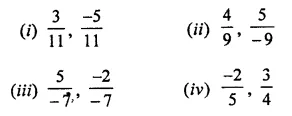Solution: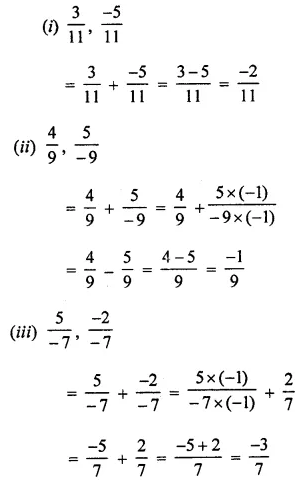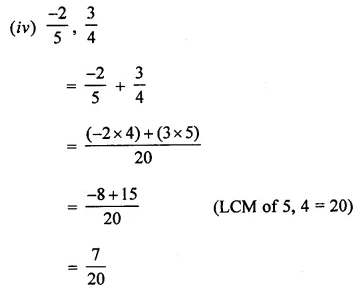Question 2.
Find the sum:Solution:Question 3.
Subtract: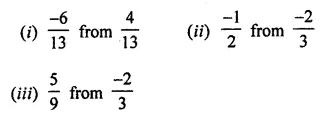Solution:Question 4.
Find:Solution: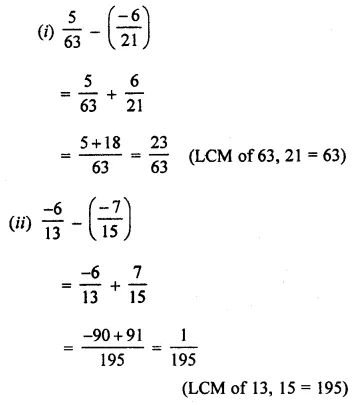Question 5.
The sum of two rational numbers is $$\frac { 2 }{ 5 }$$ if one of them is $$\frac { -4 }{ 7 }$$, find the other.
Solution: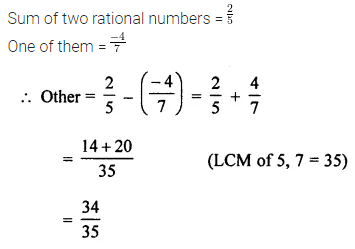Question 6.
What rational number should be added to $$\frac { -5 }{ 12 }$$ to get $$\frac { -7 }{ 8 }$$?
Solution: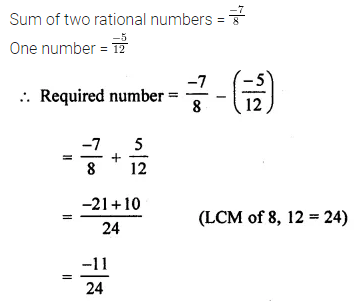Question 7.
What rational number should be subtracted from $$\frac { -2 }{ 3 }$$ to get $$\frac { -5 }{ 6 }$$?
Solution:Question 8.
Find the product:Solution: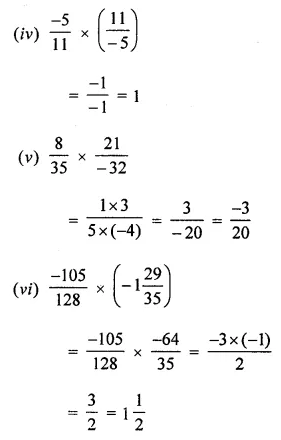Question 9.
Find the value of:Solution:Question 10.
The product of two rational numbers is $$\frac { 18 }{ 35 }$$ If one of them is $$\frac { -2 }{ 5 }$$, find the other.
Solution: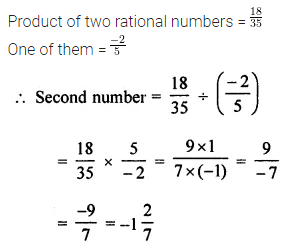Question 11.
Find the value of: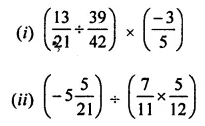Solution: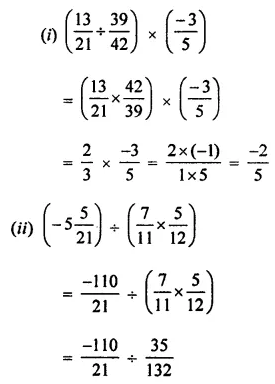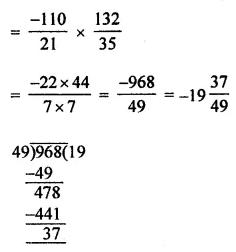Question 12.
Find the reciprocal of the following: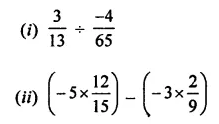Solution: